# Selina Solutions Concise Mathematics Class 6 Chapter 13: Unitary Method Exercise 13(A)

Selina Solutions Concise Mathematics Class 6 Chapter 13 Unitary Method Exercise 13(A) provides a clear cut picture of the concepts, which are essential from the exam perspective. The solutions are formulated by expert teachers in an interactive way, helping students in solving the complex questions, with ease. Practising these solutions on a daily basis improves students’ academic performance. To increase conceptual knowledge among students, Selina Solutions Concise Mathematics Class 6 Chapter 13 Unitary Method Exercise 13(A), PDF links are given here for free download

## Selina Solutions Concise Mathematics Class 6 Chapter 13: Unitary Method Exercise 13(A) Download PDF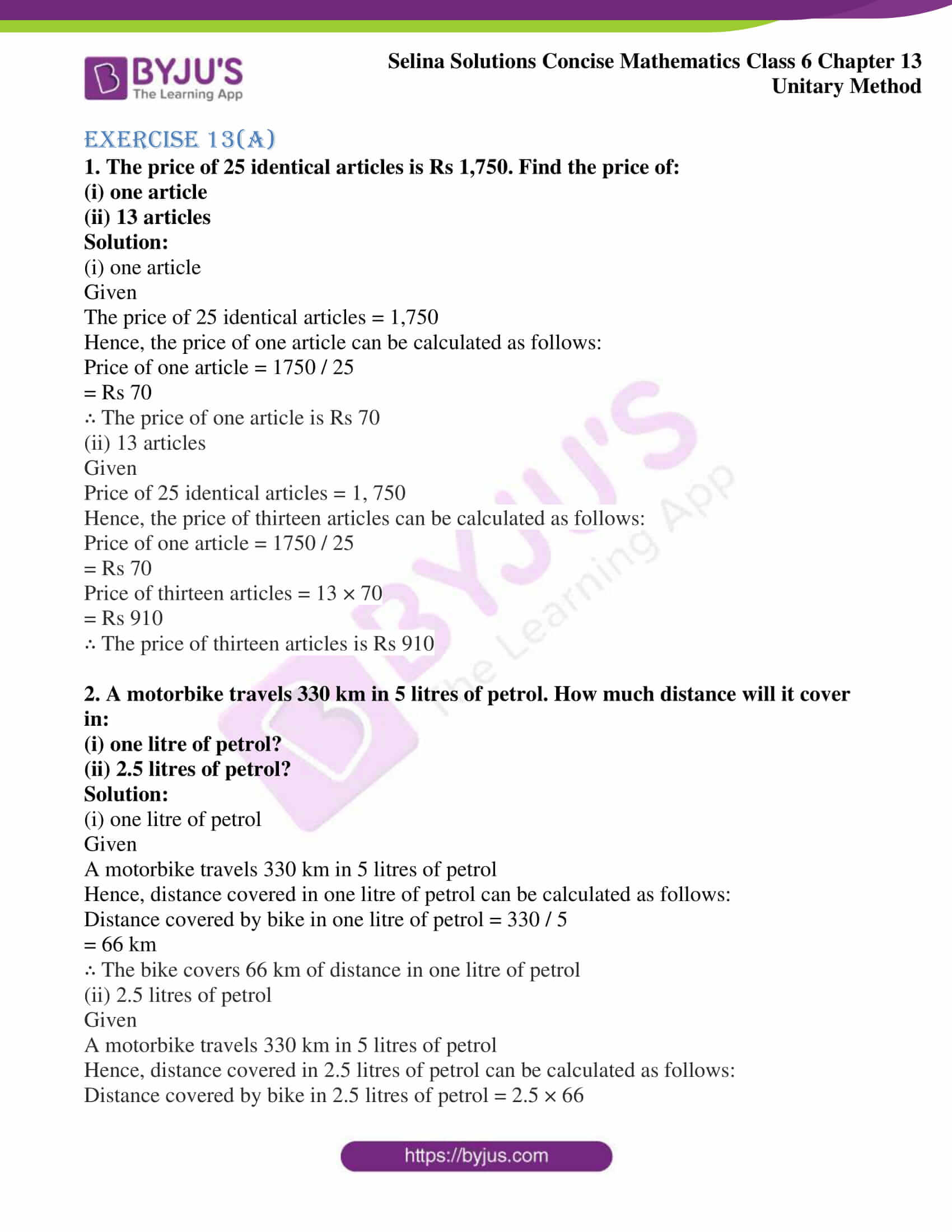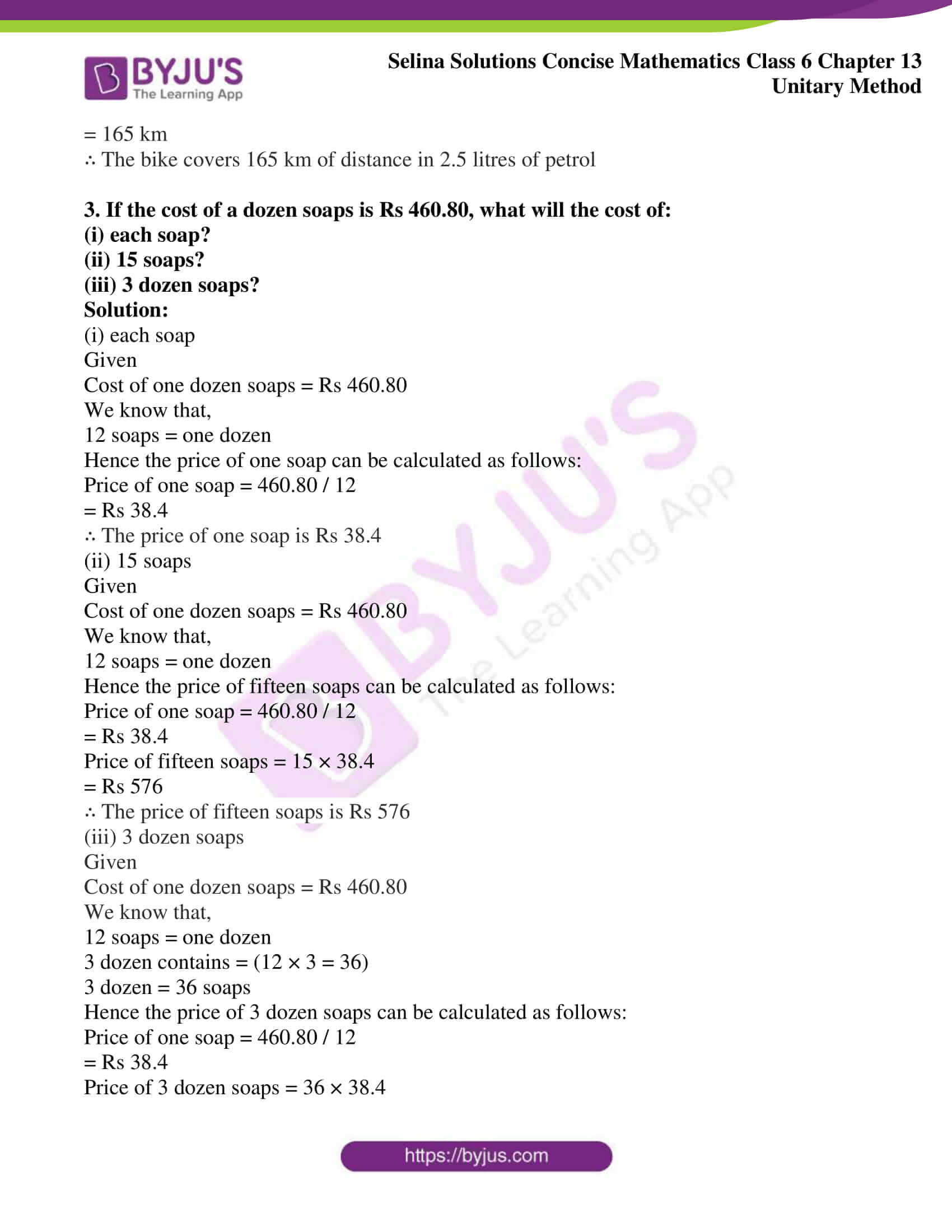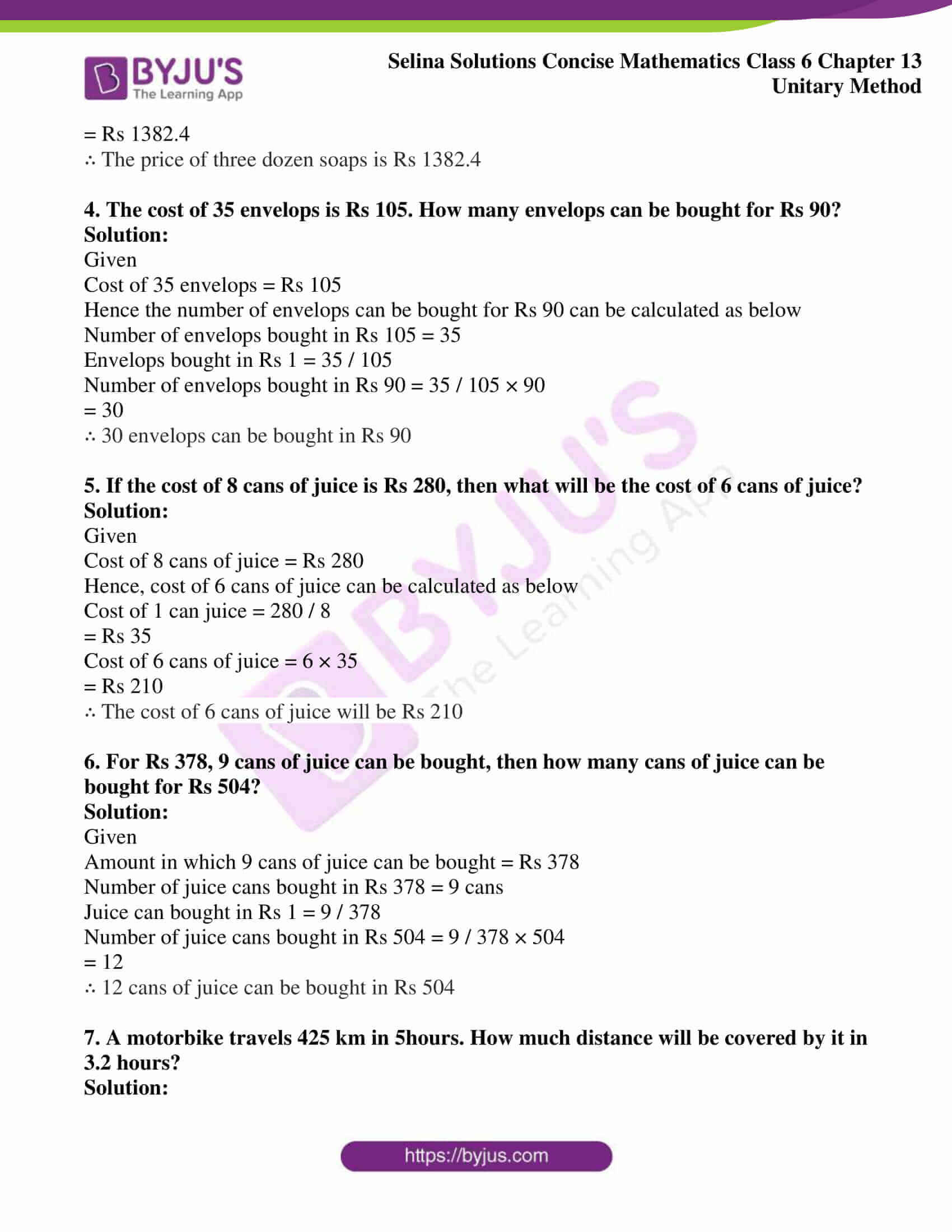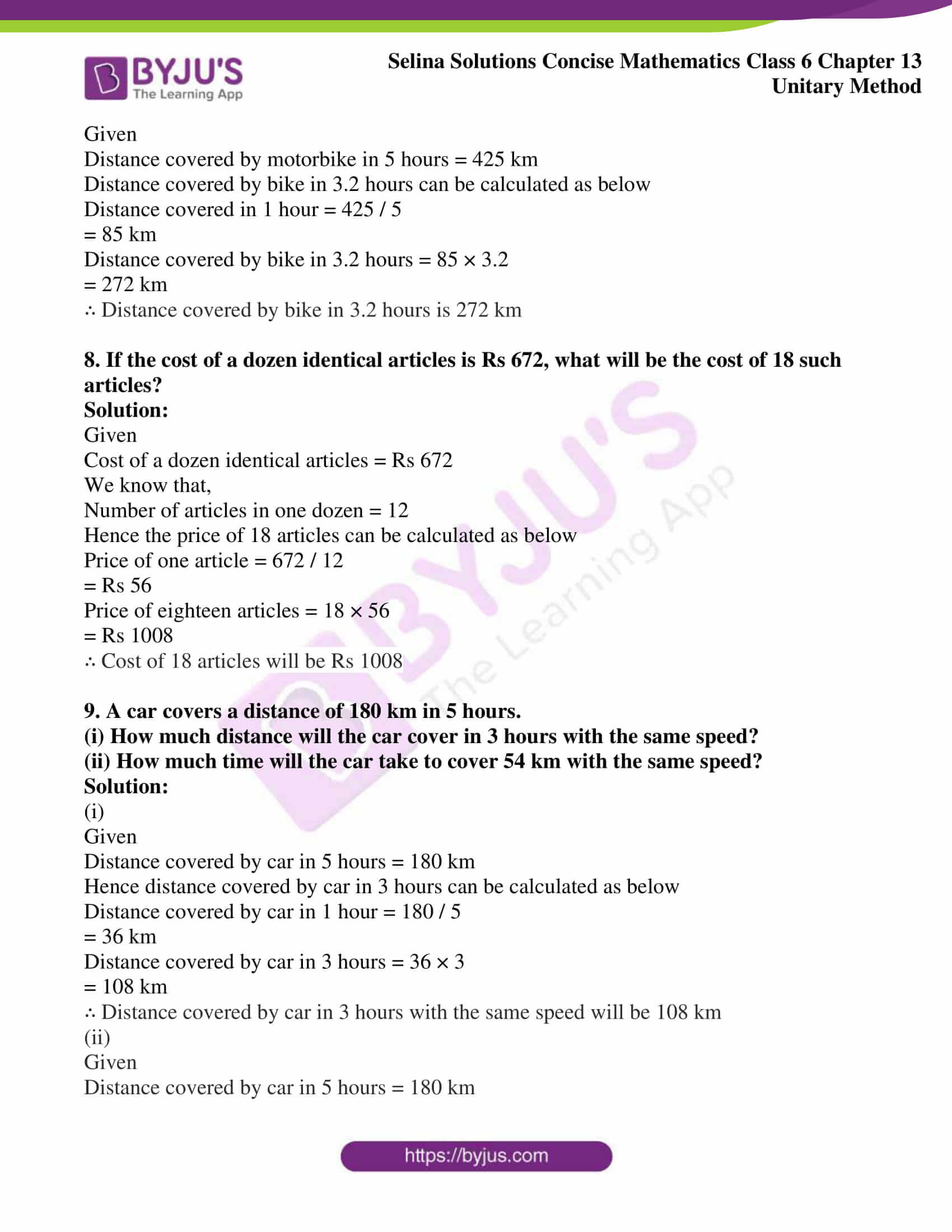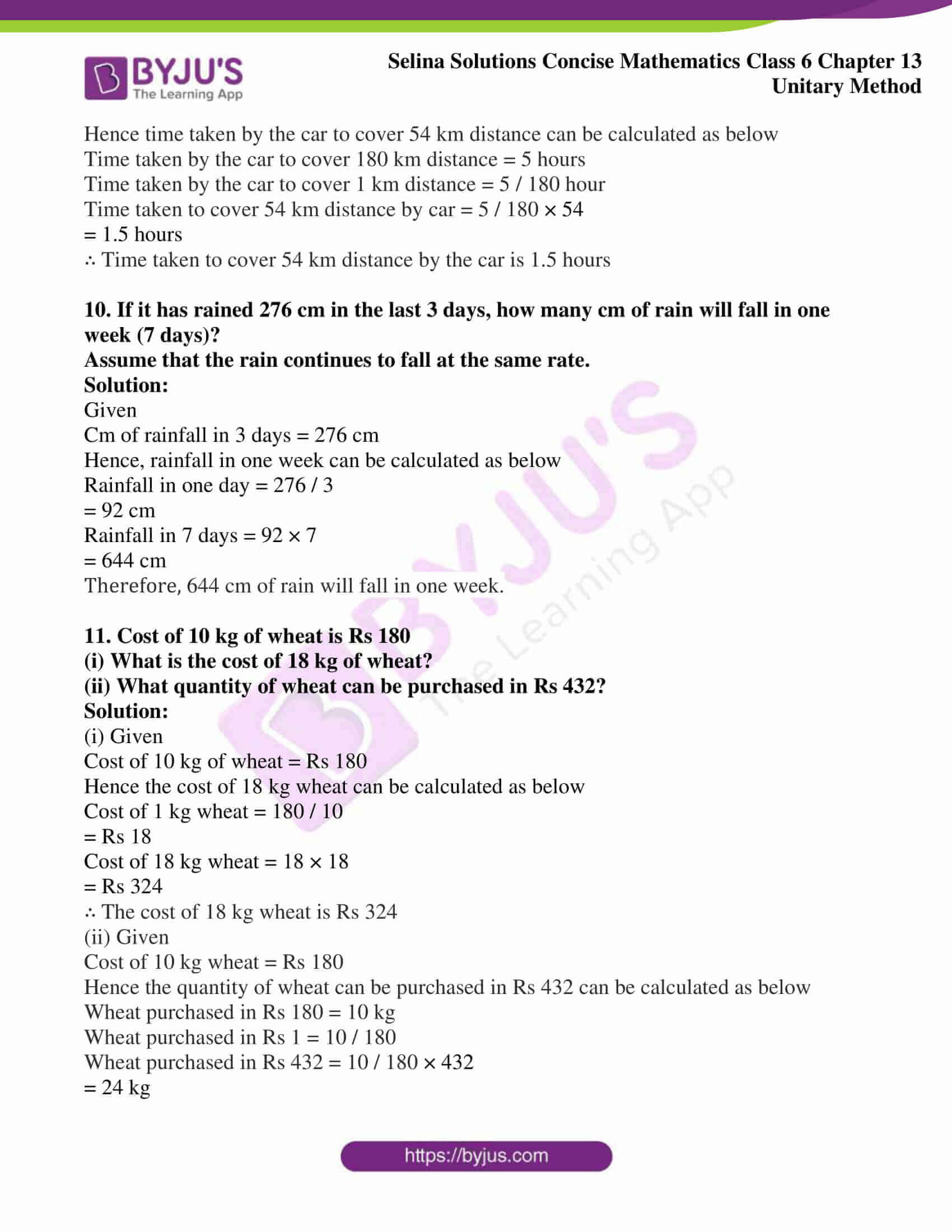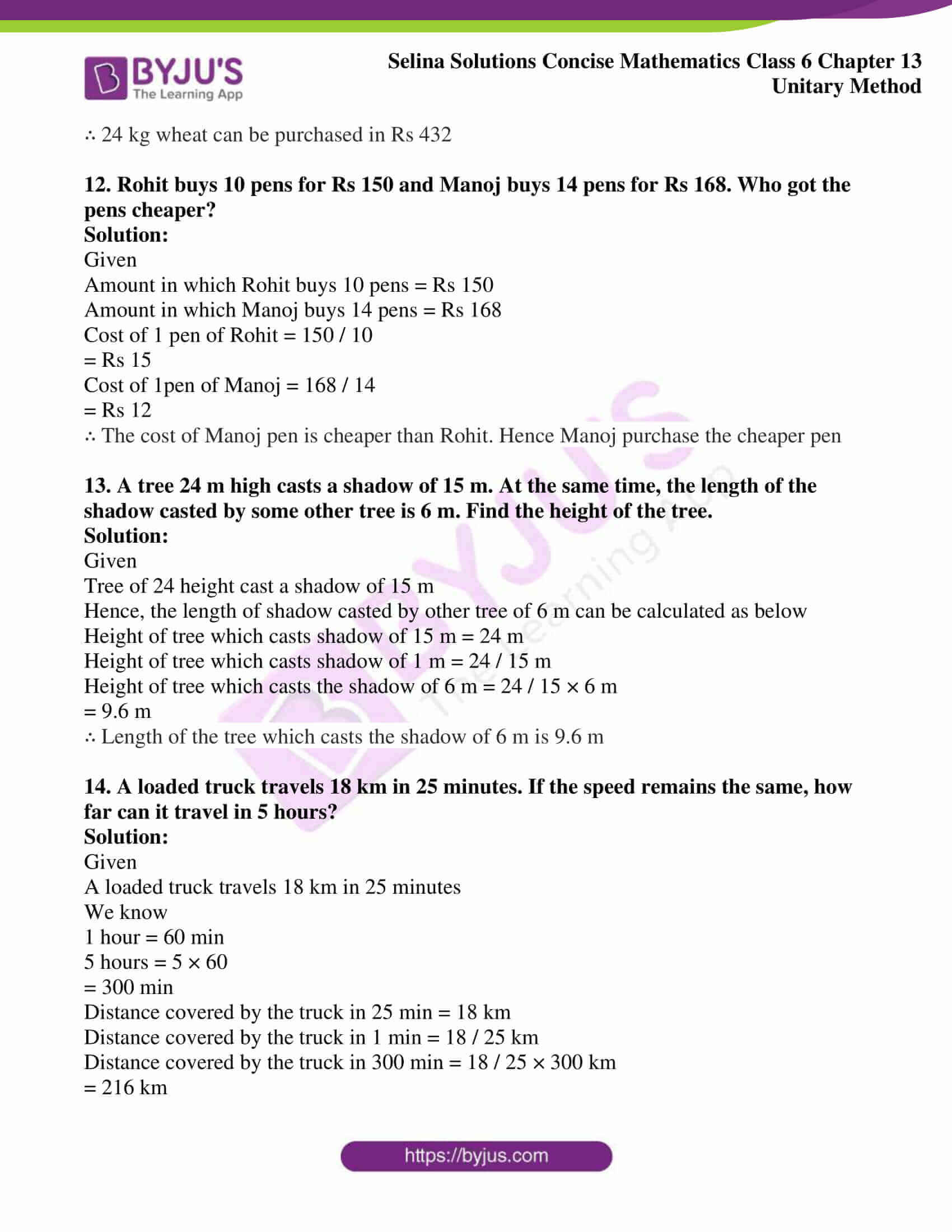### Access another exercise of Selina Solutions Concise Mathematics Class 6 Chapter 13: Unitary Method

Exercise 13(B) Solutions

### Access Selina Solutions Concise Mathematics Class 6 Chapter 13: Unitary Method Exercise 13(A)

#### Exercise 13(A)

1. The price of 25 identical articles is Rs 1,750. Find the price of:

(i) one article

(ii) 13 articles

Solution:

(i) one article

Given

The price of 25 identical articles = 1,750

Hence, the price of one article can be calculated as follows:

Price of one article = 1750 / 25

= Rs 70

∴ The price of one article is Rs 70

(ii) 13 articles

Given

Price of 25 identical articles = 1, 750

Hence, the price of thirteen articles can be calculated as follows:

Price of one article = 1750 / 25

= Rs 70

Price of thirteen articles = 13 × 70

= Rs 910

∴ The price of thirteen articles is Rs 910

2. A motorbike travels 330 km in 5 litres of petrol. How much distance will it cover in:

(i) one litre of petrol?

(ii) 2.5 litres of petrol?

Solution:

(i) one litre of petrol

Given

A motorbike travels 330 km in 5 litres of petrol

Hence, distance covered in one litre of petrol can be calculated as follows:

Distance covered by bike in one litre of petrol = 330 / 5

= 66 km

∴ The bike covers 66 km of distance in one litre of petrol

(ii) 2.5 litres of petrol

Given

A motorbike travels 330 km in 5 litres of petrol

Hence, distance covered in 2.5 litres of petrol can be calculated as follows:

Distance covered by bike in 2.5 litres of petrol = 2.5 × 66

= 165 km

∴ The bike covers 165 km of distance in 2.5 litres of petrol

3. If the cost of a dozen soaps is Rs 460.80, what will the cost of:

(i) each soap?

(ii) 15 soaps?

(iii) 3 dozen soaps?

Solution:

(i) each soap

Given

Cost of one dozen soaps = Rs 460.80

We know that,

12 soaps = one dozen

Hence the price of one soap can be calculated as follows:

Price of one soap = 460.80 / 12

= Rs 38.4

∴ The price of one soap is Rs 38.4

(ii) 15 soaps

Given

Cost of one dozen soaps = Rs 460.80

We know that,

12 soaps = one dozen

Hence the price of fifteen soaps can be calculated as follows:

Price of one soap = 460.80 / 12

= Rs 38.4

Price of fifteen soaps = 15 × 38.4

= Rs 576

∴ The price of fifteen soaps is Rs 576

(iii) 3 dozen soaps

Given

Cost of one dozen soaps = Rs 460.80

We know that,

12 soaps = one dozen

3 dozen contains = (12 × 3 = 36)

3 dozen = 36 soaps

Hence the price of 3 dozen soaps can be calculated as follows:

Price of one soap = 460.80 / 12

= Rs 38.4

Price of 3 dozen soaps = 36 × 38.4

= Rs 1382.4

∴ The price of three dozen soaps is Rs 1382.4

4. The cost of 35 envelops is Rs 105. How many envelops can be bought for Rs 90?

Solution:

Given

Cost of 35 envelops = Rs 105

Hence the number of envelops can be bought for Rs 90 can be calculated as below

Number of envelops bought in Rs 105 = 35

Envelops bought in Rs 1 = 35 / 105

Number of envelops bought in Rs 90 = 35 / 105 × 90

= 30

∴ 30 envelops can be bought in Rs 90

5. If the cost of 8 cans of juice is Rs 280, then what will be the cost of 6 cans of juice?

Solution:

Given

Cost of 8 cans of juice = Rs 280

Hence, cost of 6 cans of juice can be calculated as below

Cost of 1 can juice = 280 / 8

= Rs 35

Cost of 6 cans of juice = 6 × 35

= Rs 210

∴ The cost of 6 cans of juice will be Rs 210

6. For Rs 378, 9 cans of juice can be bought, then how many cans of juice can be bought for Rs 504?

Solution:

Given

Amount in which 9 cans of juice can be bought = Rs 378

Number of juice cans bought in Rs 378 = 9 cans

Juice can bought in Rs 1 = 9 / 378

Number of juice cans bought in Rs 504 = 9 / 378 × 504

= 12

∴ 12 cans of juice can be bought in Rs 504

7. A motorbike travels 425 km in 5hours. How much distance will be covered by it in 3.2 hours?

Solution:

Given

Distance covered by motorbike in 5 hours = 425 km

Distance covered by bike in 3.2 hours can be calculated as below

Distance covered in 1 hour = 425 / 5

= 85 km

Distance covered by bike in 3.2 hours = 85 × 3.2

= 272 km

∴ Distance covered by bike in 3.2 hours is 272 km

8. If the cost of a dozen identical articles is Rs 672, what will be the cost of 18 such articles?

Solution:

Given

Cost of a dozen identical articles = Rs 672

We know that,

Number of articles in one dozen = 12

Hence the price of 18 articles can be calculated as below

Price of one article = 672 / 12

= Rs 56

Price of eighteen articles = 18 × 56

= Rs 1008

∴ Cost of 18 articles will be Rs 1008

9. A car covers a distance of 180 km in 5 hours.

(i) How much distance will the car cover in 3 hours with the same speed?

(ii) How much time will the car take to cover 54 km with the same speed?

Solution:

(i)

Given

Distance covered by car in 5 hours = 180 km

Hence distance covered by car in 3 hours can be calculated as below

Distance covered by car in 1 hour = 180 / 5

= 36 km

Distance covered by car in 3 hours = 36 × 3

= 108 km

∴ Distance covered by car in 3 hours with the same speed will be 108 km

(ii)

Given

Distance covered by car in 5 hours = 180 km

Hence time taken by the car to cover 54 km distance can be calculated as below

Time taken by the car to cover 180 km distance = 5 hours

Time taken by the car to cover 1 km distance = 5 / 180 hour

Time taken to cover 54 km distance by car = 5 / 180 × 54

= 1.5 hours

∴ Time taken to cover 54 km distance by the car is 1.5 hours

10. If it has rained 276 cm in the last 3 days, how many cm of rain will fall in one week (7 days)?

Assume that the rain continues to fall at the same rate.

Solution:

Given

Cm of rainfall in 3 days = 276 cm

Hence, rainfall in one week can be calculated as below

Rainfall in one day = 276 / 3

= 92 cm

Rainfall in 7 days = 92 × 7

= 644 cm

Therefore, 644 cm of rain will fall in one week.

11. Cost of 10 kg of wheat is Rs 180

(i) What is the cost of 18 kg of wheat?

(ii) What quantity of wheat can be purchased in Rs 432?

Solution:

(i) Given

Cost of 10 kg of wheat = Rs 180

Hence the cost of 18 kg wheat can be calculated as below

Cost of 1 kg wheat = 180 / 10

= Rs 18

Cost of 18 kg wheat = 18 × 18

= Rs 324

∴ The cost of 18 kg wheat is Rs 324

(ii) Given

Cost of 10 kg wheat = Rs 180

Hence the quantity of wheat can be purchased in Rs 432 can be calculated as below

Wheat purchased in Rs 180 = 10 kg

Wheat purchased in Rs 1 = 10 / 180

Wheat purchased in Rs 432 = 10 / 180 × 432

= 24 kg

∴ 24 kg wheat can be purchased in Rs 432

12. Rohit buys 10 pens for Rs 150 and Manoj buys 14 pens for Rs 168. Who got the pens cheaper?

Solution:

Given

Amount in which Rohit buys 10 pens = Rs 150

Amount in which Manoj buys 14 pens = Rs 168

Cost of 1 pen of Rohit = 150 / 10

= Rs 15

Cost of 1pen of Manoj = 168 / 14

= Rs 12

∴ The cost of Manoj pen is cheaper than Rohit. Hence Manoj purchase the cheaper pen

13. A tree 24 m high casts a shadow of 15 m. At the same time, the length of the shadow casted by some other tree is 6 m. Find the height of the tree.

Solution:

Given

Tree of 24 height cast a shadow of 15 m

Hence, the length of shadow casted by other tree of 6 m can be calculated as below

Height of tree which casts shadow of 15 m = 24 m

Height of tree which casts shadow of 1 m = 24 / 15 m

Height of tree which casts the shadow of 6 m = 24 / 15 × 6 m

= 9.6 m

∴ Length of the tree which casts the shadow of 6 m is 9.6 m

14. A loaded truck travels 18 km in 25 minutes. If the speed remains the same, how far can it travel in 5 hours?

Solution:

Given

A loaded truck travels 18 km in 25 minutes

We know

1 hour = 60 min

5 hours = 5 × 60

= 300 min

Distance covered by the truck in 25 min = 18 km

Distance covered by the truck in 1 min = 18 / 25 km

Distance covered by the truck in 300 min = 18 / 25 × 300 km

= 216 km

∴ Distance travelled by the truck in 5 hours is 216 km